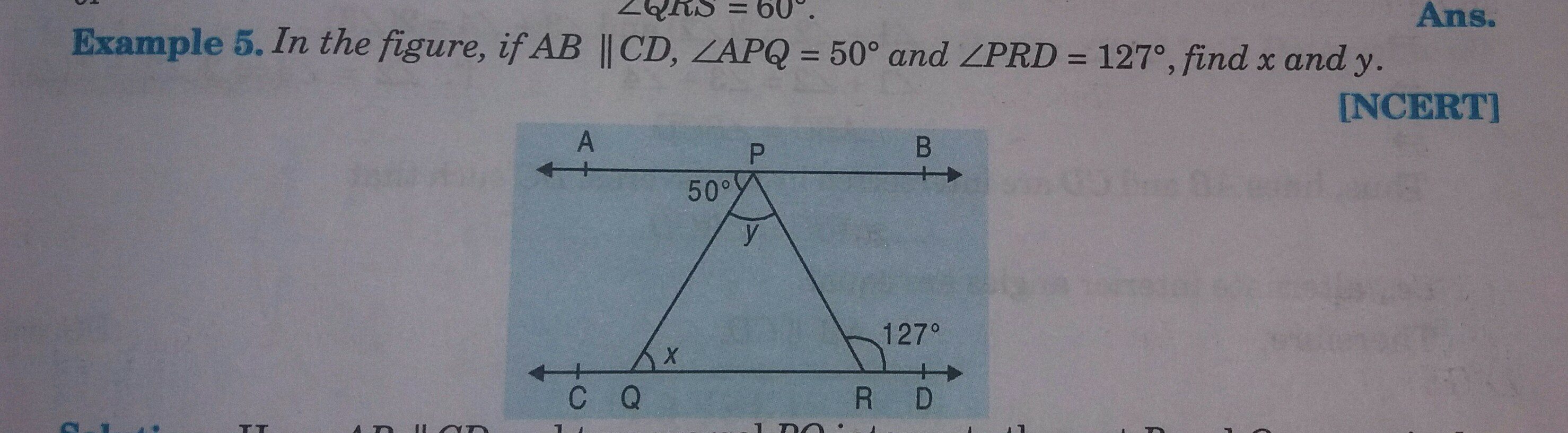"
">

# Find x and y."

Given :

AB || CD

∠APQ = 50°  and ∠PRD = 127°

To find :

We have to find the value of x and y

Solution :

AB || CD and PQ is a transversal.

$x = 50°$    (Alternate angles are equal)

We know that,

An exterior angle of a triangle is equal to the sum of the opposite interior angles.

Therefore,

$x+y = 127°$

$50°+y = 127°$

$y = 127°-50°$

$y = 77°$

The measures of x and y are 50° and 77° respectively.

Updated on: 10-Oct-2022

36 Views